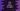# How to override toString method to print contents of a object in Java### Java example to override toString method to print contents of an object :

To print the contents of an object, we need to override toString() method of that object. In this tutorial we will learn how to override toString() method of an object and what are the benifits of doing it.

### Example :

We have two class Student.java and Main.java. Inside Main.java we will create an arraylist to contain few Student objects. Then we will iterate the list and print out the object values.

Following are the classes :

### Student.java

``````public class Student {
//1
//student name
private String studentName;

//2
//marks of the student
private int studentMarks;

//3
/**
* Constructor to create a Student object
*
* @param name  : Name of the student
* @param marks : Marks of the student
*/
public Student(String name, int marks) {
this.studentName = name;
this.studentMarks = marks;
}

//4
@Override
public String toString() {
return "Marks for " + studentName + " is " + studentMarks;
}
}
``````
1. studentName variable contains the name of the student.

2. studentMarks variable contains the marks of the student.

3. public Student(String name, int marks) is the constructor to create one Student object. We will pass the name and age of the student and save it in the object in studentName and studentMarks variables.

4. We are overriding the toString() method in this class and the return String is the combination of studentName and studentMarks.

Now, let’s take a look into the Main.java class :

### Main.java

``````import java.util.ArrayList;

public class Main {

public static void main(String[] args) {
//1
ArrayList studentList = new ArrayList();

//2

//3
for(Student s : studentList){
System.out.println(s);
}
}

}
``````
1. First we have created one ArrayList studentList that can hold objects of type Student.

2. Next, we added five different Student objects with different name and age.

3. Using a for loop, we have printout the objects. Note that we are directly passing the object to the System.out.println() .

### Output :

The above program will print the below output:

``````Marks for Alex is 35
Marks for Bryan is 45
Marks for Andy is 58
Marks for Jane is 95
Marks for Lily is 77
``````

So, the program print out the same output we have returned from the override toString() method.